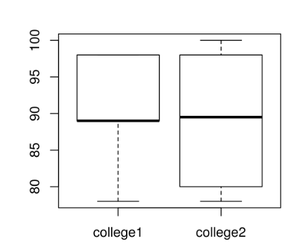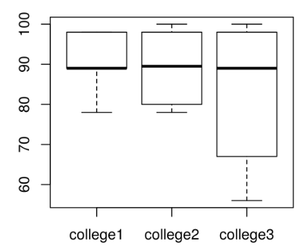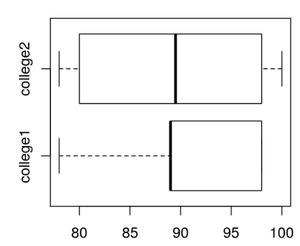Related Articles

# How to Make a Side by Side Boxplot in R ?

• Last Updated : 30 May, 2021

In this article, we are going to discuss how to create box plots side by side in the R programming language. If we want to create multiple box plots side by side then we have to specify a parameter in the above syntax i.e., beside=T. It will specify True. This will place the second, third,.,so on to next of the box plots

Syntax:

boxplot(data,beside=T)

Where,

• beside is to place bar plots side by side
• data is the combination of vector data which represents number of box plots needed to plot.

data=cbind(vector1,vector2,.,vector n)

Thus to implement this approach, first, the data to create each boxplot is initialized and then all of these are combined using cbind(). The combined data is then passed to the boxplot function along with beside parameter set to TRUE to draw them side by side.

Example 1:

## R

 `# college1 vector``college1=``c``(98,89,89.0,78,98,89)`` ` `# college2 vector``college2=``c``(88,91,100,78,98,80)`` ` `# combine two vectors using cbind``# function``college_data=``cbind``(college1,college2)`` ` `# pass this college_data to the ``# boxplot``boxplot``(college_data,beside=T)`

Output:Example 2:

## R

 `# college1 vector``college1=``c``(98,89,89.0,78,98,89)`` ` `# college2 vector``college2=``c``(88,91,100,78,98,80)`` ` `# college3 vector``college3=``c``(98,89,89.0,100,67,56)`` ` `# combine three college vectors using``# cbind function``college_data=``cbind``(college1,college2,college3)`` ` `# pass this college_data to the box plot``boxplot``(college_data,beside=T)`

Output:If we want to display the box plots side by side in a horizontal way, we can pass the parameter horizontal in the syntax.

Syntax:

boxplot(data,beside=T,horizontal=T)

Specifies the horizontal = True: arranges the plots in horizontal

If F- False, arranges in the vertical fashion

Example 3:

## R

 `# college1 vector``college1=``c``(98,89,89.0,78,98,89)`` ` `# college2 vector``college2=``c``(88,91,100,78,98,80)`` ` `# combine two college vectors ``# using cbind function``college_data=``cbind``(college1,college2)`` ` `# pass this college_data to the ``# box plot``boxplot``(college_data,beside=T,horizontal=T)`

Output:My Personal Notes arrow_drop_up